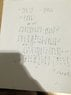# Finding a matrix W such that W^t*AW = D (D is diagonal matrix)

• zjohnson19

## Homework Statement

A = 000
010
101

Find Eigenvalues, its corresponding eigenvectors, and find a matrix W such that W^t*AW = D, where D is a diagnol matrix.(note that W^t represents the transpose of W)

## Homework Equations

Eigenvalues, Eigenvectors, diagnolization[/B]

## The Attempt at a Solution

Question 1 is to find eigenvalues. Since it is already a lower triangular matrix this was easy and I believe the eigenvalues are 1 and 0. The characteristic equation I got was (0-y)(1-y)(1-y).

Question 2 was to find the corresponding eigenvectors. if y1 = 1, then (A-1I) = -100
000
100

From that you get x1 = 0. x2 and x3 are free. So a basis for the eigenspace is (v1, v2) where
v1 = 0
1
0

v2 = 0
0
1

For y = 0, A -0 is just A. you get x2 = 0 and x1 = -x3 with x3 free. Therefore the corresponding eigenvector is
v = -1
0
1

Correct me if I'm wrong but I believe up to here I have done everything correct.

The final part of the question confuses me quite a bit . "find a matrix W such that W^t*AW = D, where D is a diagnol matrix."

I thought diagnolization is only possible if a matrix is invertible. Since A has 0 as an eigenvalue, it is not invertible. Did I get the eigenvectors wrong or is D not the diagnolization of A?

Edit: I cannot find a way to get the space formatting correct. Does anyone know how?

Last edited:

## Homework Statement

A = 000
010
101

Find Eigenvalues, its corresponding eigenvectors, and find a matrix W such that W^t*AW = D, where D is a diagnol matrix.

## Homework Equations

Eigenvalues, Eigenvectors, diagnolization[/B]

## The Attempt at a Solution

Question 1 is to find eigenvalues. Since it is already a lower triangular matrix this was easy and I believe the eigenvalues are 1 and 0. The characteristic equation I got was (0-y)(1-y)(1-y).

Question 2 was to find the corresponding eigenvectors. if y1 = 1, then (A-1I) = -100
000
100

From that you get x1 = 0. x2 and x3 are free. So a basis for the eigenspace is (v1, v2) where
v1 = 0
1
0

v2 = 0
0
0

For y = 0, A -0 is just A. you get x2 = 0 and x1 = -x3 with x3 free. Therefore the corresponding eigenvector is
v = -1
0
1

Correct me if I'm wrong but I believe up to here I have done everything correct.

The final part of the question confuses me quite a bit . "find a matrix W such that W^t*AW = D, where D is a diagnol matrix."

I thought diagnolization is only possible if a matrix is invertible. Since A has 0 as an eigenvalue, it is not invertible. Did I get the eigenvectors wrong or is D not the diagnolization of A?

Edit: I cannot find a way to get the space formatting correct. Does anyone know how?

The zero vector is never considered as an eigenvector, so your v2 is incorrect. Not only that, you want the eigenvectors to form a basis (which is possible in the diagonalizable case), and the zero vector is never part of a basis.

My bad, I had that copied down wrong(I edited it now to fix it). I had v2 as (0 0 1), not (0 0 0). So a basis for the eigenspace corresponding to lambda = 0 would be

 [ 0]
|1| , | 0|
 [ 1]

one eigenvector would be

|1|


I've tried to diagnolize the matrix and I found an P and A that work(since AP = PD).

I got p from the bases of the eigenspaces.
p =
00-1
10 0
01 1

I chose D to be the eigenvalues in the diagnol positions corresponding with its bases in p
D=
100
010
000

AP=PD so this step should be fine.

I then was hoping this would work for the final answer and I could choose P as the answer. Unfortunately the transpose of P * AP does not equal this diagnol matrix.

Anyone know where to go from here?

#### Attachments

•IMG_3887.JPG
20.9 KB · Views: 415